Courses
Courses for Kids
Free study material
Offline Centres
More

# 2 Digit Addition and SubtractionLast updated date: 29th Nov 2023
Total views: 98.1k
Views today: 0.98k## Introduction:

Kids, suppose you have 12 chocolates and your parents asked you to give 4 chocolates to your friend. Now, how many chocolates you are left with? Yes, you are right! Now, you are left with 8 chocolates. But do you know the name of the method using which you found the correct number of chocolates you are left with? We'll be doing 2-digit addition and subtraction today.

Adding two-digit integers together is known as two-digit addition. The outcome is referred to as the total of the two numbers. The practice of removing two or more numbers with two digits from one another is known as two-digit subtraction.

There are four basic operations of arithmetic, i.e., addition, subtraction, multiplication, and division. Among these, today we will be talking about the addition. Adding two whole numbers will result in the combination of the two values. Every arithmetic operation has its unique symbol. In addition, the sign is ‘+’.

For example:

What number of balls does Rishi have altogether if he has 3 green balls and 2 blue balls?

The total number of balls is $3 + 2 =5$.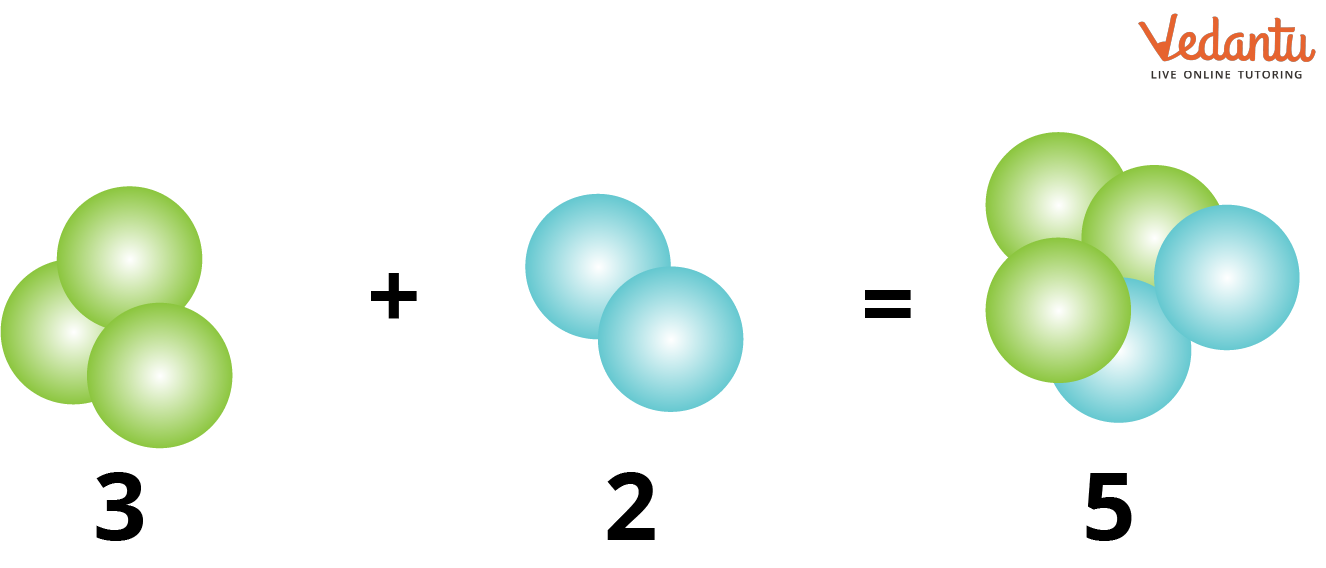Sum of $3 + 2$ balls

## What is Subtraction?

Subtraction means taking away things from a group or several things. Every arithmetic operation has its unique symbol. In subtraction, the sign is ‘-’.

Try to write the larger number first and the smaller number directly below when subtracting two numbers. After that, remove each column one at a time.

Suppose, Kriti has 10 chocolates and she gives up 2 chocolates. Then what is the total number of chocolates that Kriti has?

The total number of chocolates that Kriti has $10 - 2 = 8$.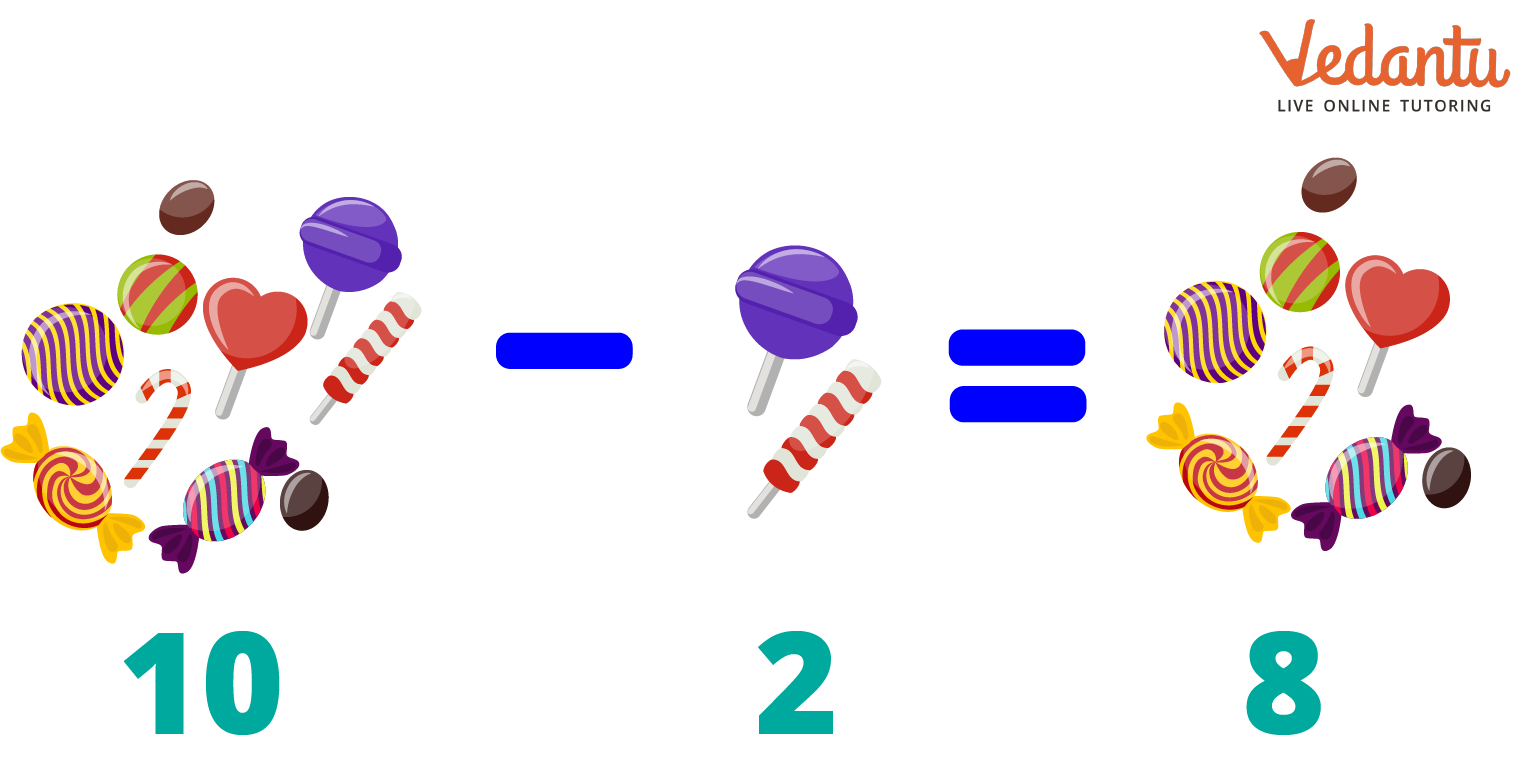Difference of $10 - 2$ chocolates

## How Does Adding and Subtracting 2-Digit Numbers Works?

In addition, the place value of the numbers is given more importance. Here, the place value is expressed as ones and tens. To add 2-digit numbers, first, we need to arrange them column-wise according to their place values. Digits are added one by one. Here, the numbers are called addends, and the answer is known as their sum.

There are two methods for regrouping, namely 2-digit addition and subtraction with regrouping. Without regrouping, 2-digit addition and subtraction are also possible.

In subtraction, the place value of the numbers is also given more importance. After subtraction, the number of things reduces or becomes less. The subtraction consists of the minuend, subtrahend, and difference.

It can be written as $Minuend{\rm{ }} - {\rm{ }}Subtrahend{\rm{ }} = {\rm{ }}Difference$.

There is a condition in the ‘regrouping’ method. Here, we have to move the extra number to the next column if the sum of the numbers in either of the columns is more than 9.

For example: Take the addition of 29 and 52

As there is a carry-over value of 1, hence, the result will be 81.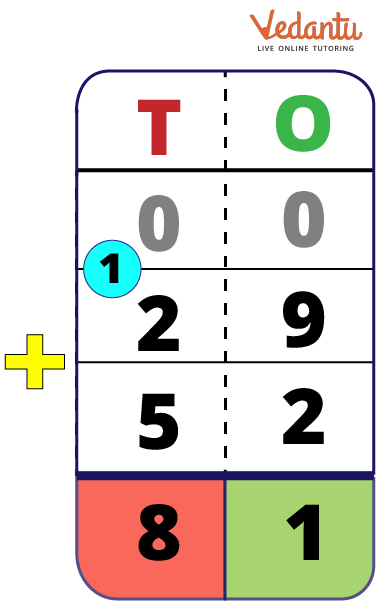Sum of $29 + 52$

• 2 Digit Addition without Regrouping:

The ‘Without regrouping’ method is very simple. Here, when the sum is less than or equal to 9, we write the number according to its place value column. It does not carry forward any number.

For example: Take the addition of 20 and 34

As there are no carry-over value, hence, the result will be 54.

## Types of Subtraction

• 2 Digit Subtraction with Regrouping:

If the number at the bottom is greater than the top, take 1 from the tens column and add it to the number at the one's position. The number at the ten's place will decrease by one after adding 1 to the one's position. Now subtract the numbers.

For example: Take the subtraction of 71 and 49

Firstly, subtract 9 from 1. Since we cannot subtract larger numbers from smaller ones, we borrow 1 from the digit that is at the ten’s place in the minuend to make the one’s digit larger.

So, 1 becomes 11, now we can subtract 9 from 11 and the result will be 2 at the one’s column.

After giving the borrow to one’s column, the digit at the ten’s column reduced to 6.

Now, subtract 4 from 6, we get 2 at the ten’s column.
So, the final difference is 22.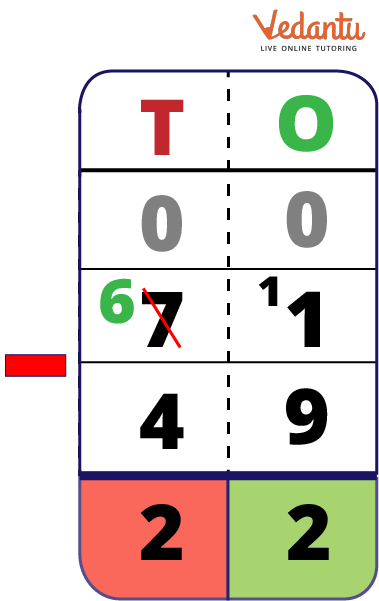Difference of 49 and 71

• 2 Digit Subtraction Without Regrouping:

The ‘Without regrouping’ method is very simple. Here, always put the greater value at the top and find the difference between the place value according to the column. It does not take carry/borrow from any number.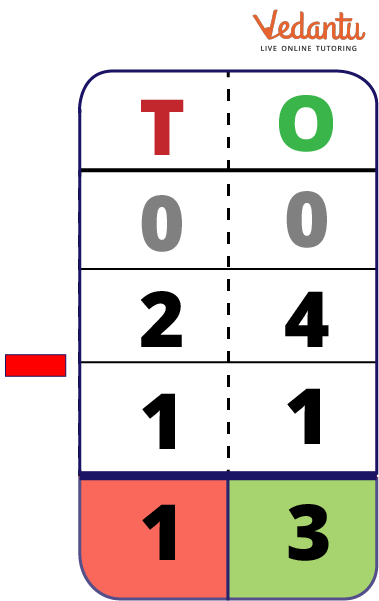Difference of $24 - 11$

## 2 Digit Addition and Subtraction Word Problems

Let us look at some :

Example 1: Suppose Sima already has 88 pens and her mother just gave her 11 more. What is Sima's total number of pens?

Solution:

According to the question, Sima already has = 88 pens and her mother gave = 11pens more.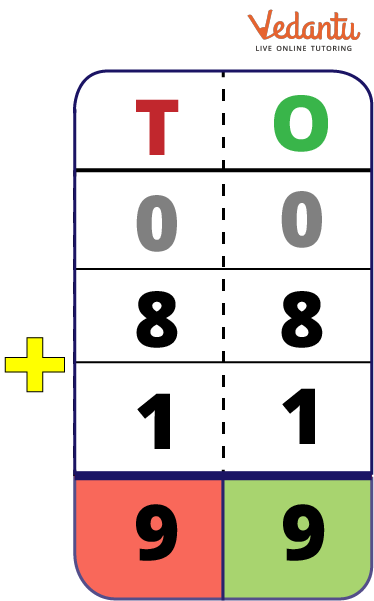Sum of $88 + 11$

Now, Sima has a total of $= 88 + 11 = 99$ pens

Therefore, the total number of pens that Sima has is 99.

Example 2: Suppose Tina already has 99 oranges. She gave up and 55 oranges . Then her father gave her 12 oranges. What is Tins's total number of oranges?

Solution:

According to the question, Tina already has = 99 oranges, she gave up =55 oranges and her father gave = 12 oranges.

First, arrange the numbers with the smaller number followed by the bigger one, $= 99 - 55 = 44$

Then, adding the given and the obtained number,

$\begin{array}{l} = 44 + 12\\ = 56\end{array}$

Hence, Tina has a total of 56 oranges.

Example 3: 54 of the 66 flowers that Rishi had were sold. Now, how many flowers does Rishi have altogether?

Solution:

According to the question, Rishi had = 66 flowers and he sold = 54 flowers.

First, arrange the numbers with the smaller number followed by the bigger one,

$\begin{array}{l} = 66 - 54\\ = 12\end{array}$

Hence, Rishi has a total of 12 flowers.

### Conclusion

Performing addition and subtraction is very crucial in our day-to-day lifestyle. It is required in every step of life. Like, when we go out shopping or to the office, schools and colleges and while playing games. We were taught addition and subtraction as kids, and we still use them regularly.

The number of items increases with addition, while the number of items decreases with subtraction. Thus, it is obvious that both are completely at odds with one another.

## FAQs on 2 Digit Addition and Subtraction

1: Explain “Addition is the opposite of Subtraction”.

Yes, addition is indeed the opposite of subtraction. In addition, we combine things together, whereas in subtraction we eliminate the items. In addition, the amount or quantity increases whereas in subtraction the amount or quantity decreases.